## Jump and run in Pygame

In this article you will learn how to implement jump and run logic in Pygame. To do so, we will implement the player logic.

You may like
Teach Your Kids to Code: Learn Python Programming at Any AgeMovement
Moving left and right is similar to the previous tutorial and simply mean changing the (x,y) position of the player. For jumping, we use a formula from classical mechanics:

`F = 1/2 * m * v^2`

Where F is the force up/down,  m is the mass of your object and v is the velocity. The velocity goes down over time because when the player jumps the velocity will not increase more in this simulation.   If the player reaches the ground, the jump ends. In Python, we set a variable isjump to indicate if the player is jumping or not. If the player is, its position will be updated according to the above formula.

Full Code:

```from pygame.locals import * import pygame import math from time import sleep   class Player: x = 10 y = 500 speed = 10   # Stores if player is jumping or not. isjump = 0   # Force (v) up and mass m. v = 8 m = 2   def moveRight(self): self.x = self.x + self.speed   def moveLeft(self): self.x = self.x - self.speed   def jump(self): self.isjump = 1   def update(self): if self.isjump: # Calculate force (F). F = 0.5 * mass * velocity^2. if self.v &gt; 0: F = ( 0.5 * self.m * (self.v*self.v) ) else: F = -( 0.5 * self.m * (self.v*self.v) )   # Change position self.y = self.y - F   # Change velocity self.v = self.v - 1   # If ground is reached, reset variables. if self.y &gt;= 500: self.y = 500 self.isjump = 0 self.v = 8     class App:   windowWidth = 800 windowHeight = 600 player = 0   def __init__(self): self._running = True self._display_surf = None self._image_surf = None self.player = Player()   def on_init(self): pygame.init() self._display_surf = pygame.display.set_mode((self.windowWidth,self.windowHeight), pygame.HWSURFACE)   pygame.display.set_caption('Pygame pythonspot.com example') self._running = True self._image_surf = pygame.image.load("pygame.png").convert()   def on_event(self, event): if event.type == QUIT: self._running = False   def on_loop(self): pass   def on_render(self): self._display_surf.fill((0,0,0)) self._display_surf.blit(self._image_surf,(self.player.x,self.player.y)) self.player.update() pygame.display.flip() sleep(0.03)   def on_cleanup(self): pygame.quit()   def on_execute(self): if self.on_init() == False: self._running = False   while( self._running ): pygame.event.pump() keys = pygame.key.get_pressed()   if (keys[K_RIGHT]): self.player.moveRight()   if (keys[K_LEFT]): self.player.moveLeft()   if (keys[K_UP]): self.player.jump()   if (keys[K_ESCAPE]): self._running = False   self.on_loop() self.on_render() self.on_cleanup()   if __name__ == "__main__" : theApp = App() theApp.on_execute()```

If you want to jump on objects, simply add them to the screen, do collision detection and reset the jump variables if collision is true.

## Simple text game with Python

In this article we will demonstrate how to create a simple guessing game.
The goal of the game is to guess the right number.

Example
An example run below: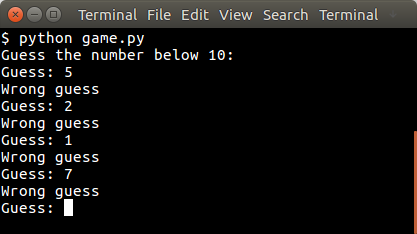Simple text game with Python

You may like

## Random number

The user will be asked to guess the random number. We first pick the random number:

```from random import randint   x = randint(1,9)```

The randint() function will pick a pseudo random number between 1 and 10. Then we have to continue until the correct number is found:

```guess = -1   print("Guess the number below 10:") while guess != x: guess = int(raw_input("Guess: "))   if guess != x: print("Wrong guess") else: print("Guessed correctly")```

## Python Guessing Game

The code below starts the game:

```from random import randint   x = randint(1,9) guess = -1   print "Guess the number below 10:" while guess != x: guess = int(raw_input("Guess: "))   if guess != x: print("Wrong guess") else: print("Guessed correctly")```

An example run:

```Guess the number below 10: Guess: 3 Wrong guess Guess: 6 Wrong guess ..```

## Snake AI in Pygame

In this article we will show you how to create basic game AI (Artificial Intelligence). This article will describe an AI for the game snake.

In this game (snake) both the computer and you play a snake, and the computer snake tries to catch you. In short: the opponent AI tries to determine and go to the destination point based on your location on the board.

You may like

Adding the computer player:
We extend the code with a new class called Computer which will be our computer player. This contains routines to draw and move the computer snake.

```class Computer: x =  y =  step = 44 direction = 0 length = 3   updateCountMax = 2 updateCount = 0   def __init__(self, length): self.length = length for i in range(0,2000): self.x.append(-100) self.y.append(-100)   # initial positions, no collision. self.x = 1*44 self.y = 4*44   def update(self):   self.updateCount = self.updateCount + 1 if self.updateCount &gt; self.updateCountMax:   # update previous positions for i in range(self.length-1,0,-1): self.x[i] = self.x[i-1] self.y[i] = self.y[i-1]   # update position of head of snake if self.direction == 0: self.x = self.x + self.step if self.direction == 1: self.x = self.x - self.step if self.direction == 2: self.y = self.y - self.step if self.direction == 3: self.y = self.y + self.step   self.updateCount = 0     def moveRight(self): self.direction = 0   def moveLeft(self): self.direction = 1   def moveUp(self): self.direction = 2   def moveDown(self): self.direction = 3   def draw(self, surface, image): for i in range(0,self.length): surface.blit(image,(self.x[i],self.y[i]))```

We then call the update and drawing method of the computer.

``` def on_loop(self): self.player.update() self.computer.update()   ....   def on_render(self): self._display_surf.fill((0,0,0)) self.player.draw(self._display_surf, self._image_surf) self.apple.draw(self._display_surf, self._apple_surf) self.computer.draw(self._display_surf, self._image_surf) pygame.display.flip()```

This will make the computer snake move and be drawn on the screen. It has the same properties as the human player.

Adding the intelligence to the computer player
Because this is a simple game, we do not need to create a complete thinking machine inside the game.  We simply need some basic  intelligence exhibited by our computer player.  Intelligence in games is often quite limited because most of the time more complexity is not neccesary or there simply is not the time available to implement clever algorithms.

The algorithm we will add will simply go to the destination. It will neglect any obstacles (the human player).

``` def target(self,dx,dy): if self.x &gt; dx: self.moveLeft()   if self.x &lt; dx: self.moveRight()   if self.x == dx: if self.y &lt; dy: self.moveDown()   if self.y &gt; dy: self.moveUp()```

Complete code
We end up with this complete code:

```from pygame.locals import * from random import randint import pygame import time   class Apple: x = 0 y = 0 step = 44   def __init__(self,x,y): self.x = x * self.step self.y = y * self.step   def draw(self, surface, image): surface.blit(image,(self.x, self.y))     class Player: x =  y =  step = 44 direction = 0 length = 3   updateCountMax = 2 updateCount = 0   def __init__(self, length): self.length = length for i in range(0,2000): self.x.append(-100) self.y.append(-100)   # initial positions, no collision. self.x = 1*44 self.x = 2*44   def update(self):   self.updateCount = self.updateCount + 1 if self.updateCount &gt; self.updateCountMax:   # update previous positions for i in range(self.length-1,0,-1): self.x[i] = self.x[i-1] self.y[i] = self.y[i-1]   # update position of head of snake if self.direction == 0: self.x = self.x + self.step if self.direction == 1: self.x = self.x - self.step if self.direction == 2: self.y = self.y - self.step if self.direction == 3: self.y = self.y + self.step   self.updateCount = 0     def moveRight(self): self.direction = 0   def moveLeft(self): self.direction = 1   def moveUp(self): self.direction = 2   def moveDown(self): self.direction = 3   def draw(self, surface, image): for i in range(0,self.length): surface.blit(image,(self.x[i],self.y[i]))       class Computer: x =  y =  step = 44 direction = 0 length = 3   updateCountMax = 2 updateCount = 0   def __init__(self, length): self.length = length for i in range(0,2000): self.x.append(-100) self.y.append(-100)   # initial positions, no collision. self.x = 1*44 self.y = 4*44   def update(self):   self.updateCount = self.updateCount + 1 if self.updateCount &gt; self.updateCountMax:   # update previous positions for i in range(self.length-1,0,-1): self.x[i] = self.x[i-1] self.y[i] = self.y[i-1]   # update position of head of snake if self.direction == 0: self.x = self.x + self.step if self.direction == 1: self.x = self.x - self.step if self.direction == 2: self.y = self.y - self.step if self.direction == 3: self.y = self.y + self.step   self.updateCount = 0     def moveRight(self): self.direction = 0   def moveLeft(self): self.direction = 1   def moveUp(self): self.direction = 2   def moveDown(self): self.direction = 3     def target(self,dx,dy): if self.x &gt; dx: self.moveLeft()   if self.x &lt; dx: self.moveRight()   if self.x == dx: if self.y &lt; dy: self.moveDown()   if self.y &gt; dy: self.moveUp()   def draw(self, surface, image): for i in range(0,self.length): surface.blit(image,(self.x[i],self.y[i]))     class Game: def isCollision(self,x1,y1,x2,y2,bsize): if x1 &gt;= x2 and x1 &lt;= x2 + bsize: if y1 &gt;= y2 and y1 &lt;= y2 + bsize: return True return False   class App:   windowWidth = 800 windowHeight = 600 player = 0 apple = 0   def __init__(self): self._running = True self._display_surf = None self._image_surf = None self._apple_surf = None self.game = Game() self.player = Player(5) self.apple = Apple(8,5) self.computer = Computer(5)   def on_init(self): pygame.init() self._display_surf = pygame.display.set_mode((self.windowWidth,self.windowHeight), pygame.HWSURFACE)   pygame.display.set_caption('Pygame pythonspot.com example') self._running = True self._image_surf = pygame.image.load("pygame.png").convert() self._apple_surf = pygame.image.load("apple.png").convert()   def on_event(self, event): if event.type == QUIT: self._running = False   def on_loop(self): self.computer.target(self.apple.x, self.apple.y) self.player.update() self.computer.update()   # does snake eat apple? for i in range(0,self.player.length): if self.game.isCollision(self.apple.x,self.apple.y,self.player.x[i], self.player.y[i],44): self.apple.x = randint(2,9) * 44 self.apple.y = randint(2,9) * 44 self.player.length = self.player.length + 1   # does computer eat apple? for i in range(0,self.player.length): if self.game.isCollision(self.apple.x,self.apple.y,self.computer.x[i], self.computer.y[i],44): self.apple.x = randint(2,9) * 44 self.apple.y = randint(2,9) * 44 #self.computer.length = self.computer.length + 1     # does snake collide with itself? for i in range(2,self.player.length): if self.game.isCollision(self.player.x,self.player.y,self.player.x[i], self.player.y[i],40): print "You lose! Collision: " print "x (" + str(self.player.x) + "," + str(self.player.y) + ")" print "x[" + str(i) + "] (" + str(self.player.x[i]) + "," + str(self.player.y[i]) + ")" exit(0)   pass   def on_render(self): self._display_surf.fill((0,0,0)) self.player.draw(self._display_surf, self._image_surf) self.apple.draw(self._display_surf, self._apple_surf) self.computer.draw(self._display_surf, self._image_surf) pygame.display.flip()   def on_cleanup(self): pygame.quit()   def on_execute(self): if self.on_init() == False: self._running = False   while( self._running ): pygame.event.pump() keys = pygame.key.get_pressed()   if (keys[K_RIGHT]): self.player.moveRight()   if (keys[K_LEFT]): self.player.moveLeft()   if (keys[K_UP]): self.player.moveUp()   if (keys[K_DOWN]): self.player.moveDown()   if (keys[K_ESCAPE]): self._running = False   self.on_loop() self.on_render()   time.sleep (50.0 / 1000.0); self.on_cleanup()   if __name__ == "__main__" : theApp = App() theApp.on_execute()```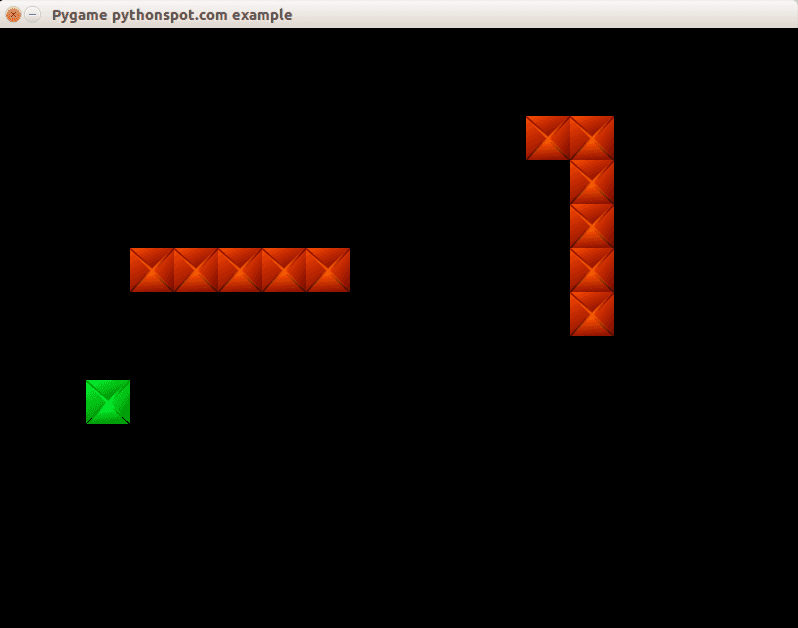python snake

Conclusion
You learned how to create a basic computer player using an very simple AI algorithm.

Next:  Learn basic sidescroller logic

## Maze in Pygame

In this tutorial you will learn how to build a maze game. The idea is simply to move around the maze with the arrow keys.

Related courses:

Getting started: Basic structure and event handling.
We define a class Player which holds the players position on the screen and the speed by which it moves. In addition we define the actions a Player instance can do (movements):

```class Player: x = 10 y = 10 speed = 1   def moveRight(self): self.x = self.x + self.speed   def moveLeft(self): self.x = self.x - self.speed   def moveUp(self): self.y = self.y - self.speed   def moveDown(self): self.y = self.y + self.speed```

A player object can be created and variables can be modified using the movement methods. We link those methods to the events. In Pygame we can get non-blocking keyboard input using this code: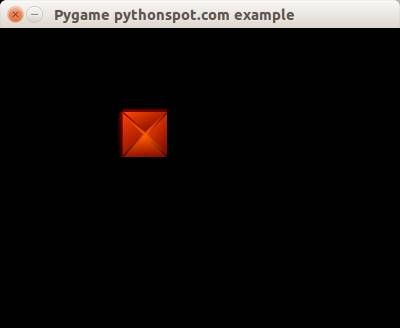pygame

Creating the maze

We define a matrix of NxM to represent the positions of the maze blocks. In this matrix the element 1 represents the presence of a block and element 0 represents the absence.

```class Maze: def __init__(self): self.M = 10 self.N = 8 self.maze = [ 1,1,1,1,1,1,1,1,1,1, 1,0,0,0,0,0,0,0,0,1, 1,0,0,0,0,0,0,0,0,1, 1,0,1,1,1,1,1,1,0,1, 1,0,1,0,0,0,0,0,0,1, 1,0,1,0,1,1,1,1,0,1, 1,0,0,0,0,0,0,0,0,1, 1,1,1,1,1,1,1,1,1,1,]```

We have this complete code to draw the maze:

```from pygame.locals import * import pygame   class Player: x = 44 y = 44 speed = 1   def moveRight(self): self.x = self.x + self.speed   def moveLeft(self): self.x = self.x - self.speed   def moveUp(self): self.y = self.y - self.speed   def moveDown(self): self.y = self.y + self.speed   class Maze: def __init__(self): self.M = 10 self.N = 8 self.maze = [ 1,1,1,1,1,1,1,1,1,1, 1,0,0,0,0,0,0,0,0,1, 1,0,0,0,0,0,0,0,0,1, 1,0,1,1,1,1,1,1,0,1, 1,0,1,0,0,0,0,0,0,1, 1,0,1,0,1,1,1,1,0,1, 1,0,0,0,0,0,0,0,0,1, 1,1,1,1,1,1,1,1,1,1,]   def draw(self,display_surf,image_surf): bx = 0 by = 0 for i in range(0,self.M*self.N): if self.maze[ bx + (by*self.M) ] == 1: display_surf.blit(image_surf,( bx * 44 , by * 44))   bx = bx + 1 if bx > self.M-1: bx = 0 by = by + 1     class App:   windowWidth = 800 windowHeight = 600 player = 0   def __init__(self): self._running = True self._display_surf = None self._image_surf = None self._block_surf = None self.player = Player() self.maze = Maze()   def on_init(self): pygame.init() self._display_surf = pygame.display.set_mode((self.windowWidth,self.windowHeight), pygame.HWSURFACE)   pygame.display.set_caption('Pygame pythonspot.com example') self._running = True self._image_surf = pygame.image.load("player.png").convert() self._block_surf = pygame.image.load("block.png").convert()   def on_event(self, event): if event.type == QUIT: self._running = False   def on_loop(self): pass   def on_render(self): self._display_surf.fill((0,0,0)) self._display_surf.blit(self._image_surf,(self.player.x,self.player.y)) self.maze.draw(self._display_surf, self._block_surf) pygame.display.flip()   def on_cleanup(self): pygame.quit()   def on_execute(self): if self.on_init() == False: self._running = False   while( self._running ): pygame.event.pump() keys = pygame.key.get_pressed()   if (keys[K_RIGHT]): self.player.moveRight()   if (keys[K_LEFT]): self.player.moveLeft()   if (keys[K_UP]): self.player.moveUp()   if (keys[K_DOWN]): self.player.moveDown()   if (keys[K_ESCAPE]): self._running = False   self.on_loop() self.on_render() self.on_cleanup()   if __name__ == "__main__" : theApp = App() theApp.on_execute()```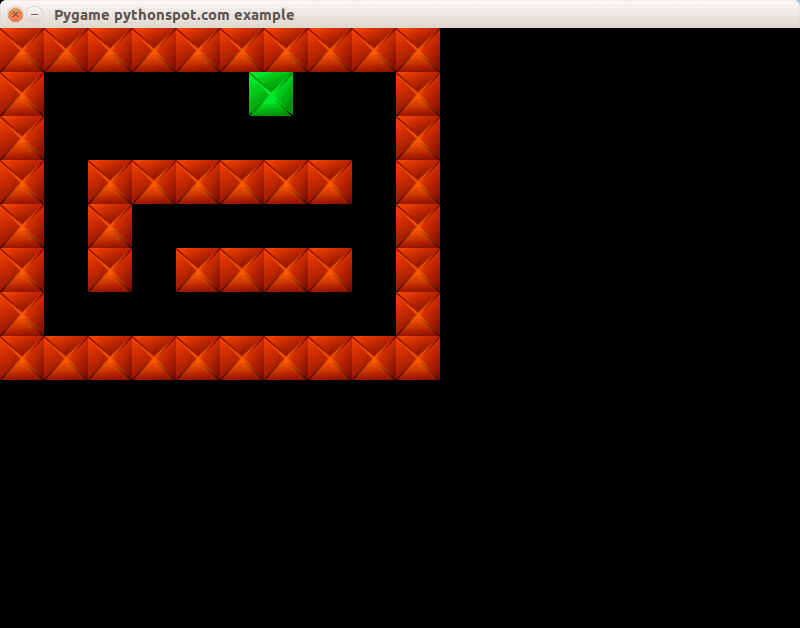maze

Concluding
You learned how to create a 2d maze in Python. Now you may want to add collision detection which we showed in our previous tutorial. Because we already explained that concept we will not go over it again 🙂

Next tutorial: AI with Pygame

## Snake with Pygame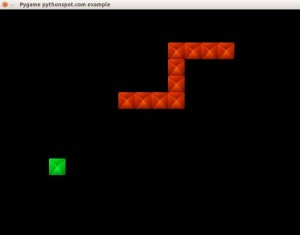In this tutorial you will learn how to build the game snake.  The game is an arcade game and it has very simple logic, which is why it is an ideal example to demonstrate how to build games with Pygame.

The player is represented as snake, which grows if it eats an apple. The goal of the game is to eat as many apples as possible without colliding into yourself.  This is very easy in the early phase of the game but is increasingly more difficult as the length of the snake grows.

Related courses:

Getting started: Basic structure and event handling.
We define a class Player which holds the players position on the screen and the speed by which it moves. In addition we define the actions a Player instance can do (movements):

```class Player: x = 10 y = 10 speed = 1   def moveRight(self): self.x = self.x + self.speed   def moveLeft(self): self.x = self.x - self.speed   def moveUp(self): self.y = self.y - self.speed   def moveDown(self): self.y = self.y + self.speed```

A player object can be created and variables can be modified using the movement methods.
We link those methods to the events. In Pygame we can get non-blocking keyboard input using this code:

``` pygame.event.pump() keys = pygame.key.get_pressed()   if (keys[K_RIGHT]): print "Right arrow pressed."```

The complete code gives us the ability to move the player across the screen:

```from pygame.locals import * import pygame   class Player: x = 10 y = 10 speed = 1   def moveRight(self): self.x = self.x + self.speed   def moveLeft(self): self.x = self.x - self.speed   def moveUp(self): self.y = self.y - self.speed   def moveDown(self): self.y = self.y + self.speed   class App:   windowWidth = 800 windowHeight = 600 player = 0   def __init__(self): self._running = True self._display_surf = None self._image_surf = None self.player = Player()   def on_init(self): pygame.init() self._display_surf = pygame.display.set_mode((self.windowWidth,self.windowHeight), pygame.HWSURFACE)   pygame.display.set_caption('Pygame pythonspot.com example') self._running = True self._image_surf = pygame.image.load("pygame.png").convert()   def on_event(self, event): if event.type == QUIT: self._running = False   def on_loop(self): pass   def on_render(self): self._display_surf.fill((0,0,0)) self._display_surf.blit(self._image_surf,(self.player.x,self.player.y)) pygame.display.flip()   def on_cleanup(self): pygame.quit()   def on_execute(self): if self.on_init() == False: self._running = False   while( self._running ): pygame.event.pump() keys = pygame.key.get_pressed()   if (keys[K_RIGHT]): self.player.moveRight()   if (keys[K_LEFT]): self.player.moveLeft()   if (keys[K_UP]): self.player.moveUp()   if (keys[K_DOWN]): self.player.moveDown()   if (keys[K_ESCAPE]): self._running = False   self.on_loop() self.on_render() self.on_cleanup()   if __name__ == "__main__" : theApp = App() theApp.on_execute()```

You can now move the block around the screen with the arrow keys.pygame example. Move the block around the screen

Building the player (snake)
The player controls a snake which has an initial length. This snake is always moving and changes the direction it moves when pressing an arrow key. To do so, update the player class:

```class Player: x = 0 y = 0 speed = 32 direction = 0   def update(self): if self.direction == 0: self.x = self.x + self.speed if self.direction == 1: self.x = self.x - self.speed if self.direction == 2: self.y = self.y - self.speed if self.direction == 3: self.y = self.y + self.speed   def moveRight(self): self.direction = 0   def moveLeft(self): self.direction = 1   def moveUp(self): self.direction = 2   def moveDown(self): self.direction = 3```

and don’t forget to add a delay to the game loop.

```import time ... time.sleep (100.0 / 1000.0);```

This starts to act more as a snake, but it does not have a base length yet. We keep track of the snakes old positions and move the head of the snake.  We also moved the drawing method into the snake. Copy the code and you will have a moving snake:

```from pygame.locals import * import pygame import time   class Player: x = [] y = [] step = 44 direction = 0 length = 3   updateCountMax = 2 updateCount = 0   def __init__(self, length): self.length = length for i in range(0,length): self.x.append(0) self.y.append(0)   def update(self):   self.updateCount = self.updateCount + 1 if self.updateCount &gt; self.updateCountMax:   # update previous positions for i in range(self.length-1,0,-1): print "self.x[" + str(i) + "] = self.x[" + str(i-1) + "]" self.x[i] = self.x[i-1] self.y[i] = self.y[i-1]   # update position of head of snake if self.direction == 0: self.x = self.x + self.step if self.direction == 1: self.x = self.x - self.step if self.direction == 2: self.y = self.y - self.step if self.direction == 3: self.y = self.y + self.step   self.updateCount = 0     def moveRight(self): self.direction = 0   def moveLeft(self): self.direction = 1   def moveUp(self): self.direction = 2   def moveDown(self): self.direction = 3   def draw(self, surface, image): for i in range(0,self.length): surface.blit(image,(self.x[i],self.y[i]))     class App:   windowWidth = 800 windowHeight = 600 player = 0   def __init__(self): self._running = True self._display_surf = None self._image_surf = None self.player = Player(10)   def on_init(self): pygame.init() self._display_surf = pygame.display.set_mode((self.windowWidth,self.windowHeight), pygame.HWSURFACE)   pygame.display.set_caption('Pygame pythonspot.com example') self._running = True self._image_surf = pygame.image.load("pygame.png").convert()   def on_event(self, event): if event.type == QUIT: self._running = False   def on_loop(self): self.player.update() pass   def on_render(self): self._display_surf.fill((0,0,0)) self.player.draw(self._display_surf, self._image_surf) pygame.display.flip()   def on_cleanup(self): pygame.quit()   def on_execute(self): if self.on_init() == False: self._running = False   while( self._running ): pygame.event.pump() keys = pygame.key.get_pressed()   if (keys[K_RIGHT]): self.player.moveRight()   if (keys[K_LEFT]): self.player.moveLeft()   if (keys[K_UP]): self.player.moveUp()   if (keys[K_DOWN]): self.player.moveDown()   if (keys[K_ESCAPE]): self._running = False self.on_loop() self.on_render()   time.sleep (50.0 / 1000.0); self.on_cleanup()   if __name__ == "__main__" : theApp = App() theApp.on_execute()```

Result: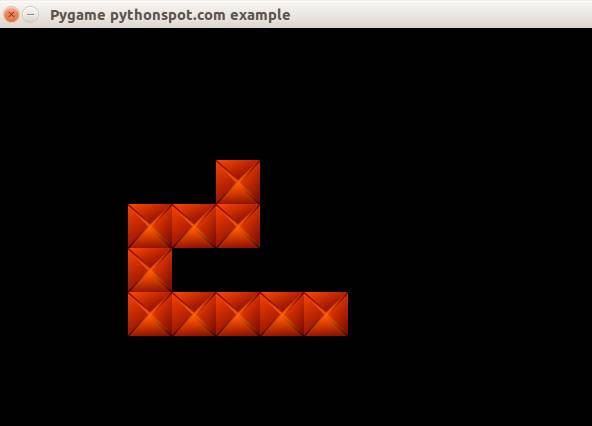python snake

Game logic
The snake game has some rules:

• If the snake eats an apple, the apple moves to a new position.
• If the snake eats an apple, the snakes length grows.
• If a snake collapses with itself, game over.

We start by creating a new class that enables us to create apples:

```class Apple: x = 0 y = 0 step = 44   def __init__(self,x,y): self.x = x * self.step self.y = y * self.step   def draw(self, surface, image): surface.blit(image,(self.x, self.y))```

For simplicity sake, we display the apples as green cubes.  We have this basic code, but it does not do a lot more than moving the snake and showing the apple:

```from pygame.locals import * import pygame import time   class Apple: x = 0 y = 0 step = 44   def __init__(self,x,y): self.x = x * self.step self.y = y * self.step   def draw(self, surface, image): surface.blit(image,(self.x, self.y))     class Player: x = [] y = [] step = 44 direction = 0 length = 3   updateCountMax = 2 updateCount = 0   def __init__(self, length): self.length = length for i in range(0,length): self.x.append(0) self.y.append(0)   def update(self):   self.updateCount = self.updateCount + 1 if self.updateCount > self.updateCountMax:   # update previous positions for i in range(self.length-1,0,-1): print "self.x[" + str(i) + "] = self.x[" + str(i-1) + "]" self.x[i] = self.x[i-1] self.y[i] = self.y[i-1]   # update position of head of snake if self.direction == 0: self.x = self.x + self.step if self.direction == 1: self.x = self.x - self.step if self.direction == 2: self.y = self.y - self.step if self.direction == 3: self.y = self.y + self.step   self.updateCount = 0     def moveRight(self): self.direction = 0   def moveLeft(self): self.direction = 1   def moveUp(self): self.direction = 2   def moveDown(self): self.direction = 3   def draw(self, surface, image): for i in range(0,self.length): surface.blit(image,(self.x[i],self.y[i]))     class App:   windowWidth = 800 windowHeight = 600 player = 0 apple = 0   def __init__(self): self._running = True self._display_surf = None self._image_surf = None self._apple_surf = None self.player = Player(10) self.apple = Apple(5,5)   def on_init(self): pygame.init() self._display_surf = pygame.display.set_mode((self.windowWidth,self.windowHeight), pygame.HWSURFACE)   pygame.display.set_caption('Pygame pythonspot.com example') self._running = True self._image_surf = pygame.image.load("pygame.png").convert() self._apple_surf = pygame.image.load("apple.png").convert()   def on_event(self, event): if event.type == QUIT: self._running = False   def on_loop(self): self.player.update() pass   def on_render(self): self._display_surf.fill((0,0,0)) self.player.draw(self._display_surf, self._image_surf) self.apple.draw(self._display_surf, self._apple_surf) pygame.display.flip()   def on_cleanup(self): pygame.quit()   def on_execute(self): if self.on_init() == False: self._running = False   while( self._running ): pygame.event.pump() keys = pygame.key.get_pressed()   if (keys[K_RIGHT]): self.player.moveRight()   if (keys[K_LEFT]): self.player.moveLeft()   if (keys[K_UP]): self.player.moveUp()   if (keys[K_DOWN]): self.player.moveDown()   if (keys[K_ESCAPE]): self._running = False   self.on_loop() self.on_render()   time.sleep (50.0 / 1000.0); self.on_cleanup()   if __name__ == "__main__" : theApp = App() theApp.on_execute()```

We have thus to add the game logic. To know if the snakes position matches the apples position, we have to do collision detection. This simply means veryfing that the cordinate of the snake are intersecting with the coordinates of the apple.  We create a new method to do that:

``` def isCollision(self,x1,y1,x2,y2,bsize): if x1 >= x2 and x1 <= x2 + bsize: if y1 >= y2 and y1 <= y2 + bsize: return True return False```

It will return True if the coordinates (x1,y1) are intersection with (x2,y2) given its block size bsize. We call this method to determine if the snake collides with the apple. We need to check this for the entire snake and not only for the head, because we do not want the new position of the apple to be somewhere on the snake. We use the same isCollision method to determine if the snake collides with itself (= lose game).

Full source:

```from pygame.locals import * from random import randint import pygame import time   class Apple: x = 0 y = 0 step = 44   def __init__(self,x,y): self.x = x * self.step self.y = y * self.step   def draw(self, surface, image): surface.blit(image,(self.x, self.y))     class Player: x =  y =  step = 44 direction = 0 length = 3   updateCountMax = 2 updateCount = 0   def __init__(self, length): self.length = length for i in range(0,2000): self.x.append(-100) self.y.append(-100)   # initial positions, no collision. self.x = 1*44 self.x = 2*44   def update(self):   self.updateCount = self.updateCount + 1 if self.updateCount > self.updateCountMax:   # update previous positions for i in range(self.length-1,0,-1): self.x[i] = self.x[i-1] self.y[i] = self.y[i-1]   # update position of head of snake if self.direction == 0: self.x = self.x + self.step if self.direction == 1: self.x = self.x - self.step if self.direction == 2: self.y = self.y - self.step if self.direction == 3: self.y = self.y + self.step   self.updateCount = 0     def moveRight(self): self.direction = 0   def moveLeft(self): self.direction = 1   def moveUp(self): self.direction = 2   def moveDown(self): self.direction = 3   def draw(self, surface, image): for i in range(0,self.length): surface.blit(image,(self.x[i],self.y[i]))   class Game: def isCollision(self,x1,y1,x2,y2,bsize): if x1 >= x2 and x1 <= x2 + bsize: if y1 >= y2 and y1 <= y2 + bsize: return True return False   class App:   windowWidth = 800 windowHeight = 600 player = 0 apple = 0   def __init__(self): self._running = True self._display_surf = None self._image_surf = None self._apple_surf = None self.game = Game() self.player = Player(3) self.apple = Apple(5,5)   def on_init(self): pygame.init() self._display_surf = pygame.display.set_mode((self.windowWidth,self.windowHeight), pygame.HWSURFACE)   pygame.display.set_caption('Pygame pythonspot.com example') self._running = True self._image_surf = pygame.image.load("block.jpg").convert() self._apple_surf = pygame.image.load("block.jpg").convert()   def on_event(self, event): if event.type == QUIT: self._running = False   def on_loop(self): self.player.update()   # does snake eat apple? for i in range(0,self.player.length): if self.game.isCollision(self.apple.x,self.apple.y,self.player.x[i], self.player.y[i],44): self.apple.x = randint(2,9) * 44 self.apple.y = randint(2,9) * 44 self.player.length = self.player.length + 1     # does snake collide with itself? for i in range(2,self.player.length): if self.game.isCollision(self.player.x,self.player.y,self.player.x[i], self.player.y[i],40): print("You lose! Collision: ") print("x (" + str(self.player.x) + "," + str(self.player.y) + ")") print("x[" + str(i) + "] (" + str(self.player.x[i]) + "," + str(self.player.y[i]) + ")") exit(0)   pass   def on_render(self): self._display_surf.fill((0,0,0)) self.player.draw(self._display_surf, self._image_surf) self.apple.draw(self._display_surf, self._apple_surf) pygame.display.flip()   def on_cleanup(self): pygame.quit()   def on_execute(self): if self.on_init() == False: self._running = False   while( self._running ): pygame.event.pump() keys = pygame.key.get_pressed()   if (keys[K_RIGHT]): self.player.moveRight()   if (keys[K_LEFT]): self.player.moveLeft()   if (keys[K_UP]): self.player.moveUp()   if (keys[K_DOWN]): self.player.moveDown()   if (keys[K_ESCAPE]): self._running = False   self.on_loop() self.on_render()   time.sleep (50.0 / 1000.0); self.on_cleanup()   if __name__ == "__main__" : theApp = App() theApp.on_execute()```

Conclusion:

You learned how to create the game snake in Python along with concepts such as collision detection, image loading and event handling. Many things could be added to this little toy game but this serves as a very simple example. 🙂

Next tutorial

## Game development with PygamePygame Python

Welcome to the first tutorial of the series: Building games with Pygame. Games you create with Pygame can be run on any machine that supports Python, including Windows, Linux and Mac OS.

In this tutorial we will explain the fundamental of building a game with Pygame. We’ll start of with the basics and will teach you how to create the basic framework. In the next tutorials you will learn how to make certain types of games.

You may like

PyGame introduction
You’ll end up with a program similar to the one on the right:

A game always starts in an order similar to this (pseudo code):

```initialize() while running(): game_logic() get_input() update_screen() deinitialize()```

The game starts with initialization. All graphics are loaded, sounds are loaded, levels are loaded and any data that needs to be loaded. The game continues running until it receives a quit event. In this game loop we update the game, get input and update the screen. Depending on the game the implementation widely varies, but this fundamental structure is common in all games.

In Pygame we define this as:

```import pygame from pygame.locals import *   class App:   windowWidth = 640 windowHeight = 480 x = 10 y = 10   def __init__(self): self._running = True self._display_surf = None self._image_surf = None   def on_init(self): pygame.init() self._display_surf = pygame.display.set_mode((self.windowWidth,self.windowHeight), pygame.HWSURFACE) self._running = True self._image_surf = pygame.image.load("pygame.png").convert()   def on_event(self, event): if event.type == QUIT: self._running = False   def on_loop(self): pass   def on_render(self): self._display_surf.blit(self._image_surf,(self.x,self.y)) pygame.display.flip()   def on_cleanup(self): pygame.quit()   def on_execute(self): if self.on_init() == False: self._running = False   while( self._running ): for event in pygame.event.get(): self.on_event(event) self.on_loop() self.on_render() self.on_cleanup()   if __name__ == "__main__" : theApp = App() theApp.on_execute()```

The Pygame program starts with the constructor __init__(). Once that is finished on_execute() is called. This method runs the game:  it updates the events, updates the screen.  Finally, the game is deinitialized using on_cleanup().

In the initialiasation phase we set the screen resolution and start the Pygame library:

``` def on_init(self): pygame.init() self._display_surf = pygame.display.set_mode((self.windowWidth,self.windowHeight), pygame.HWSURFACE)```

We also load the image.

` self._image_surf = pygame.image.load("pygame.png").convert()`

This does not draw the image to the screen, that occurs in on_render().

``` def on_render(self): self._display_surf.blit(self._image_surf,(self.x,self.y)) pygame.display.flip()```

The blit method draws the image (image_surf) to the coordinate (x,y). In Pygame the coordinates start from (0,0) top left to (wind0wWidth, windowHeight).  The method  call pygame.display.flip() updates the screen.

Continue the next tutorial and learn how to add game logic and build games 🙂